# 基于BP神经网络的海洋压力模拟设备PID控制PID Control of Marine Pressure Simulator Based on BP Neural Network

• 全文下载: PDF(2458KB)    PP.155-163   DOI: 10.12677/IaE.2019.73022
• 下载量: 171  浏览量: 705   国家自然科学基金支持

Marine pressure simulation is of great significance to Marine resource development. Ocean pres-sure simulators help in the development of deep water. This paper introduces a new type of Marine pressure simulator with double motors and pistons. After introducing the structure and working principle, the mathematical model of the system is established. Pressure simulator has the charac-teristics of nonlinearity, strong time-variability, strong interference and strong coupling. Tradi-tional PID cannot meet the control requirements. Therefore, BP neural network PID control pres-sure simulation equipment is proposed. After the feasibility is verified by simulation experiment in matlab, the experimental platform is built for experimental verification. The experimental results show that BP neural network PID control can overcome the defect of traditional PID control to some extent and improve the performance of pressure simulation equipment. In the step pressure experiment of 1 MPa, the response time of 1.5 s and the relative overshoot of 8.7% were reduced and the anti-interference property was enhanced.

1. 引言

2. 结构原理和数学模型建立

2.1. 机械结构和原理1.伺服电机；2.联轴器；3.丝杆；4.活塞式液压缸；5.进给系统；6.压力缸；7.压力传感器；8.小电机进给系统；9.工控机

Figure 1. Structure of pressure simulator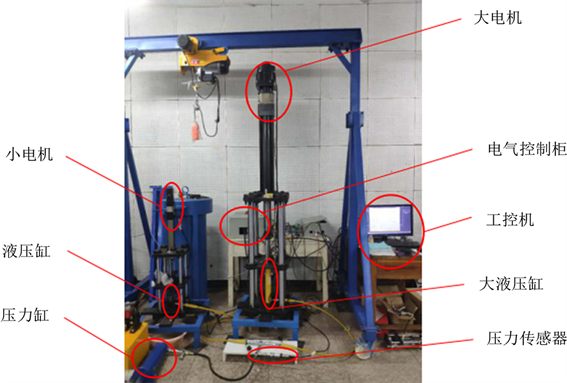Figure 2. Pressure simulation experiment platform

2.2. 数学模型建立

${G}_{1}\left(s\right)=\frac{{K}_{p1}{K}_{i}{K}_{t}\left(1+{T}_{P1}s\right)}{{T}_{P1}\left(s+{K}_{i}\right)\left(Js+{K}_{t}{K}_{u}\right){s}^{2}+{K}_{P}{K}_{P1}{K}_{i}{K}_{t}\left(1+{T}_{P1}s\right)}$ (1)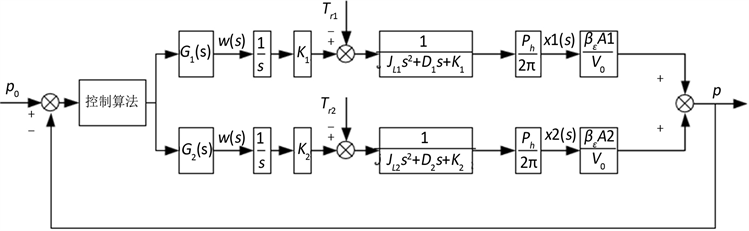Figure 3. Mathematical model of system control

3. 控制算法及仿真

3.1. 传统PID

$U\left(t\right)={T}_{P}\left[e\left(t\right)+\frac{1}{{T}_{I}}\underset{0}{\overset{t}{\int }}e\left(t\right)dt+\frac{{T}_{D}de\left(t\right)}{dt}\right]$ (2)

3.2. BP神经网络PID控制器的设计Figure 4. BP neural network PID control principle

BP神经网络是基于BP算法(误差逆传播算法)的一种多层前馈神经网络，其结构包括输入层、隐含层和输出层，相同层之间神经元不连接，相邻层之间通过权值连接 。原理图如图4，当神经网络工作时，样本信息由输入层传入网络中，在网络正向计算出各个节点的输出值。再比较网络输出值与期望值的误差，然后由输出层开始反向计算到隐藏层，按照一定算法规律调整各连接层之间的权值，重复上述步骤直至误差减小到期望以内。

BP神经网络PID控制器的设计应从确定神经网络层数、每层神经元个数以及每个神经元的激活函数开始。根据史春朝论文中刚络的层数理论：只需一个包含足够多神经元的隐层，赴欧称前馈网络就能以任意精度逼近任意复杂度的连续函数 。理论上隐藏层数的增加可以提高精度和降低误差，但同时也会使网络变得复杂，增加学习时间 。所以本文神经网络设计为具有输入层、一个隐藏层和输出层即可满足本控制器的要求。其中输入层有3个节点，偏差e、实际输出y、期望输出x，输出层也有3个节点，即PID控制器的三个参数 ${K}_{i}$${K}_{p}$${K}_{d}$ 。隐藏层的神经元数影响着网络的精度，若神经元数太少，则无法训练；如果刚好够用，虽然可以训练，但会出现网络的泛化能力差，拟合精度会降低；当神经元数太多时，会导致网络结构复杂，出现过拟合现象 。所以隐藏层节点的数量较为重要，但目前没有具体的理论指导隐藏层节点数目的确定，在实际运用中通常使用“试错法”得到。本项目中，经过多次试验，得到隐藏层节点数为5时效果较为理想。所以，神经网络结构选取为3-5-3，如图5所示。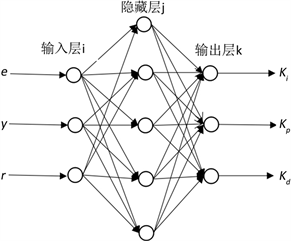Figure 5. Schematic diagram of three-layer neural network

${x}_{j}=\sum {x}_{i}{w}_{ij},\text{\hspace{0.17em}}{y}_{j}=f\left({x}_{j}\right)$ (3)

${x}_{k}=\sum {x}_{j}{w}_{jk},\text{\hspace{0.17em}}{y}_{k}=g\left({x}_{k}\right)$ (4)

$E=\frac{1}{2}{\sum \left({y}_{k}-{d}_{k}\right)}^{2}$ (5)

$\Delta {w}_{jk}=-a\frac{\partial E}{\partial {w}_{jk}},\text{\hspace{0.17em}}\Delta {w}_{ij}=-a\frac{\partial E}{\partial {w}_{ij}}$ (6)

${w}_{ij}={w}_{ij}+\Delta w$ (7)

$f\left(x\right)=\frac{{e}^{x}-{e}^{-x}}{{e}^{x}+{e}^{-x}}$ (8)

$g\left(x\right)=\frac{{e}^{x}}{{e}^{x}+{e}^{-x}}$ (9)

3.3. 仿真实验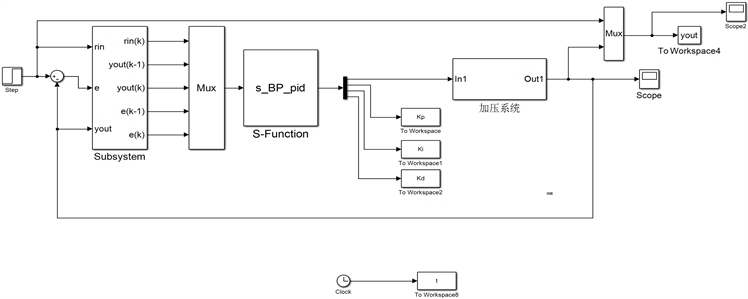Figure 6. Simulation model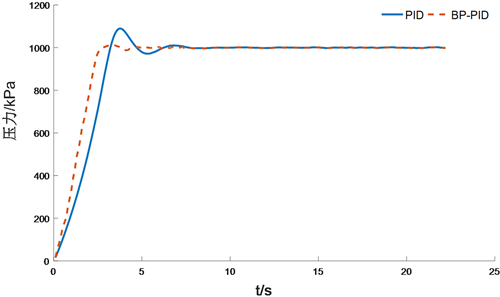Figure 7. 1 MPa step pressure simulation experiment

4. 实验

4.1. 阶跃压力实验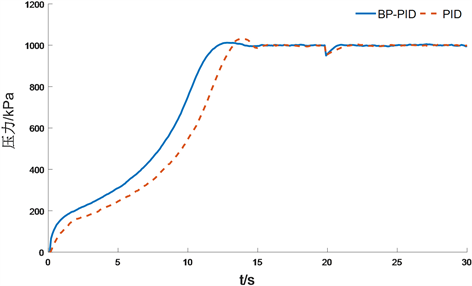Figure 8. 1MPa step pressure experimentTable 1. Pressure response in PID and neural PID control

4.2. 动态压力实验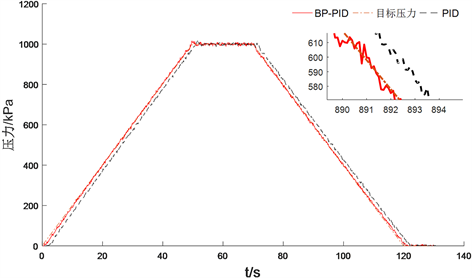Figure 9. Trapezoidal pressure simulation experiment

5. 结论

  张兰婷, 翟璐, 王波. 充分发挥海洋在高质量发展中的战略要地作用[N]. 中国海洋报, 2018.  Gonzaiez, F.I., Milburn, H.B., Bernard, R.N., et al. (1998) Deep-Ocean Assessment and Reporting of Tsunamis (DART): Brief Overview and Status Report. Proceedings of the International Workshop on Tsuami Disater Mitigation, Tokyo, 19-22 January 1998, 118-129.  吴建美, 王勇, 刘正士. 高精度深海压力模拟装置的分析[J]. 机床与液压, 2015, 43(10): 73-75.  于凯本, 杨涛, 孟庆健, 王晓阳, 李正光. 大型深海高压环境模拟系统筒体疲劳破坏分析[J]. 中国海洋大学学报(自然科学版), 2017, 47(s1): 117-122.  潘成梁. 海洋深度模拟器的电液控制系统研究[D]: [硕士学位论文]. 武汉: 华中科技大学, 2012.  吴珏斐. 某型压力筒控制系统的设计与仿真[D]: [硕士学位论文]. 武汉: 华中科技大学, 2011.  耿立平. 大型压力筒的压力控制试验系统研制[D]: [硕士学位论文]. 武汉: 华中科技大学, 2012.  朱骏, 王淮阳, 王勇. 一种新型深海压力传感器的设计与系统仿真研究[J]. 合肥工业大学学报(自然科学版), 2017, 40(2): 150-153.  王同建. 液压传动与控制[M]. 北京: 机械工业出版社, 2014: 134-136.  李维嘉, 李绍安, 罗兴桂. 高精度压力筒试验系统[J]. 中国造船, 2008, 49(1): 72-76.  唐元恒, 王占勇, 邹刚, 等. 基于BP神经网络的PID控制器的设计及模拟研究[J]. 电子测试, 2017(9): 37+15.  史春朝. BP神经网络算法的改进及其在PID控制中的应用研究[D]: [硕士学位论文]. 天津: 天津大学, 2006.  盛贤君, 姜涛, 王杰, 刘宁. 基于BP神经网络的速度前馈PID控制器设计[J]. 计算机应用, 2015, 35(s2): 134-137.  文增红. 基于BP神经网络PID的控制系统研究[J]. 电脑知识与技术, 2013, 9(28): 6375-6377+6405.  Yulunay, Y. and Senalp, E.T. (2008) A Fuzzy Neural Network Model to Forecast the Percent Cloud Coverage and Cloud Top Temperature Maps. Annales Geophysicae, 26, 35-38. https://doi.org/10.5194/angeo-26-3945-2008  杨艺, 虎恩典. 基于S函数的BP神经网络PID控制器及Simulink仿真[J]. 电子设计工程, 2014, 22(4): 29-31+35.